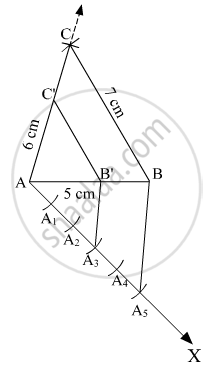# Construct a Triangle with Sides 5 Cm, 6 Cm, and 7 Cm and Then Another Triangle Whose Sides Are 3 5 of the Corresponding Sides of the First Triangle. - Mathematics

Sum

Construct a triangle with sides 5 cm, 6 cm, and 7 cm and then another triangle whose sides are 3/5 of the corresponding sides of the first triangle.

#### Solution1. Draw a line AB = 5 cm and draw a ray from A and taking A as centre cut an arc at C of 6 cm and taking B as centre cut an arc of 7 cm at C
2. Draw AX such that ∠BAX is an acute angle.
3. Cut 5 equal arcs AA1, A1A2, A2A3, A3A4, and A4A5.
4. Join A5 to B and draw a line through A3 parallel to A5B which meets AB at B'.
Here,  AB' = 3/5AB
5. Now draw a line through B' parallel to BC which joins AC at C'.
Here, B'C' = 3/5BC  "and"  AC' = 3/5AC
Thus, AB'C' is the required triangle.

Concept: Similarity of Triangles
Is there an error in this question or solution?